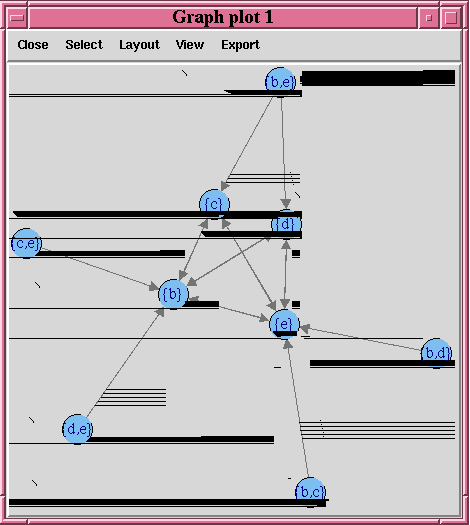# 4.2.2 Building an Association Rules Model

The `ore.odmAssocRules` function implements the apriori algorithm to find frequent itemsets and generate an association model. It finds the co-occurrence of items in large volumes of transactional data such as in the case of market basket analysis. An association rule identifies a pattern in the data in which the appearance of a set of items in a transactional record implies another set of items. The groups of items used to form rules must pass a minimum threshold according to how frequently they occur (the support of the rule) and how often the consequent follows the antecedent (the confidence of the rule). Association models generate all rules that have support and confidence greater than user-specified thresholds. The apriori algorithm is efficient, and scales well with respect to the number of transactions, number of items, and number of itemsets and rules produced.

The `formula` specification has the `form ~ terms`, where `terms` is a series of column names to include in the analysis. Multiple column names are specified using `+` between column names. Use `~ .` if all columns in data should be used for model building. To exclude columns, use `-` before each column name to exclude. Functions can be applied to the items in `terms` to realize transformations.

The `ore.odmAssocRules` function accepts data in the following forms:

• Transactional data

• Multi-record case data using item id and item value

• Relational data

For examples of specifying the forms of data and for information on the arguments of the function, invoke `help(ore.odmAssocRules)`.

The function `rules` returns an object of class `ore.rules`, which specifies a set of association rules. You can pull an `ore.rules` object into memory in a local R session by using `ore.pull`. The local in-memory object is of class `rules` defined in the `arules` package. See `help(ore.rules)`.

The function `itemsets` returns an object of class `ore.itemsets`, which specifies a set of itemsets. You can pull an `ore.itemsets` object into memory in a local R session by using `ore.pull`. The local in-memory object is of class `itemsets` defined in the `arules` package. See `help(ore.itemsets)`.

Example 4-8 Using the ore.odmAssocRules Function

This example builds an association model on a transactional data set. The packages `arules` and `arulesViz` are required to pull the resulting rules and itemsets into the client R session memory and be visualized. The graph of the rules appears in Figure 4-1.

```# Load the arules and arulesViz packages.
library(arules)
library(arulesViz)
# Create some transactional data.
id <- c(1, 1, 1, 2, 2, 2, 2, 3, 3, 3, 3)
item <- c("b", "d", "e", "a", "b", "c", "e", "b", "c", "d", "e")
# Push the data to the database as an ore.frame object.
transdata_of <- ore.push(data.frame(ID = id, ITEM = item))
# Build a model with specifications.
ar.mod1 <- ore.odmAssocRules(~., transdata_of, case.id.column = "ID",
item.id.column = "ITEM", min.support = 0.6, min.confidence = 0.6,
max.rule.length = 3)
# Generate itemsets and rules of the model.
itemsets <- itemsets(ar.mod1)
rules <- rules(ar.mod1)
# Convert the rules to the rules object in arules package.
rules.arules <- ore.pull(rules)
inspect(rules.arules)
# Convert itemsets to the itemsets object in arules package.
itemsets.arules <- ore.pull(itemsets)
inspect(itemsets.arules)
# Plot the rules graph.
plot(rules.arules, method = "graph", interactive = TRUE)
```
Listing for Example 4-8
```R> # Load the arules and arulesViz packages.
R> library(arules)
R> library(arulesViz)
R> # Create some transactional data.
R> id <- c(1, 1, 1, 2, 2, 2, 2, 3, 3, 3, 3)
R> item <- c("b", "d", "e", "a", "b", "c", "e", "b", "c", "d", "e")
R> # Push the data to the database as an ore.frame object.
R> transdata_of <- ore.push(data.frame(ID = id, ITEM = item))
R> # Build a model with specifications.
R> ar.mod1 <- ore.odmAssocRules(~., transdata_of, case.id.column = "ID",
+             item.id.column = "ITEM", min.support = 0.6, min.confidence = 0.6,
+             max.rule.length = 3)
R> # Generate itemsets and rules of the model.
R> itemsets <- itemsets(ar.mod1)
R> rules <- rules(ar.mod1)
R> # Convert the rules to the rules object in arules package.
R> rules.arules <- ore.pull(rules)
R> inspect(rules.arules)
lhs    rhs   support confidence lift
1  {b} => {e} 1.0000000  1.0000000    1
2  {e} => {b} 1.0000000  1.0000000    1
3  {c} => {e} 0.6666667  1.0000000    1
4  {d,
e} => {b} 0.6666667  1.0000000    1
5  {c,
e} => {b} 0.6666667  1.0000000    1
6  {b,
d} => {e} 0.6666667  1.0000000    1
7  {b,
c} => {e} 0.6666667  1.0000000    1
8  {d} => {b} 0.6666667  1.0000000    1
9  {d} => {e} 0.6666667  1.0000000    1
10 {c} => {b} 0.6666667  1.0000000    1
11 {b} => {d} 0.6666667  0.6666667    1
12 {b} => {c} 0.6666667  0.6666667    1
13 {e} => {d} 0.6666667  0.6666667    1
14 {e} => {c} 0.6666667  0.6666667    1
15 {b,
e} => {d} 0.6666667  0.6666667    1
16 {b,
e} => {c} 0.6666667  0.6666667    1
R> # Convert itemsets to the itemsets object in arules package.
R> itemsets.arules <- ore.pull(itemsets)
R> inspect(itemsets.arules)
items   support
1  {b}   1.0000000
2  {e}   1.0000000
3  {b,
e}   1.0000000
4  {c}   0.6666667
5  {d}   0.6666667
6  {b,
c}   0.6666667
7  {b,
d}   0.6666667
8  {c,
e}   0.6666667
9  {d,
e}   0.6666667
10 {b,
c,
e}   0.6666667
11 {b,
d,
e}   0.6666667

R> # Plot the rules graph.
R> plot(rules.arules, method = "graph", interactive = TRUE)
```

Figure 4-1 A Visual Demonstration of the Association RulesDescription of "Figure 4-1 A Visual Demonstration of the Association Rules"